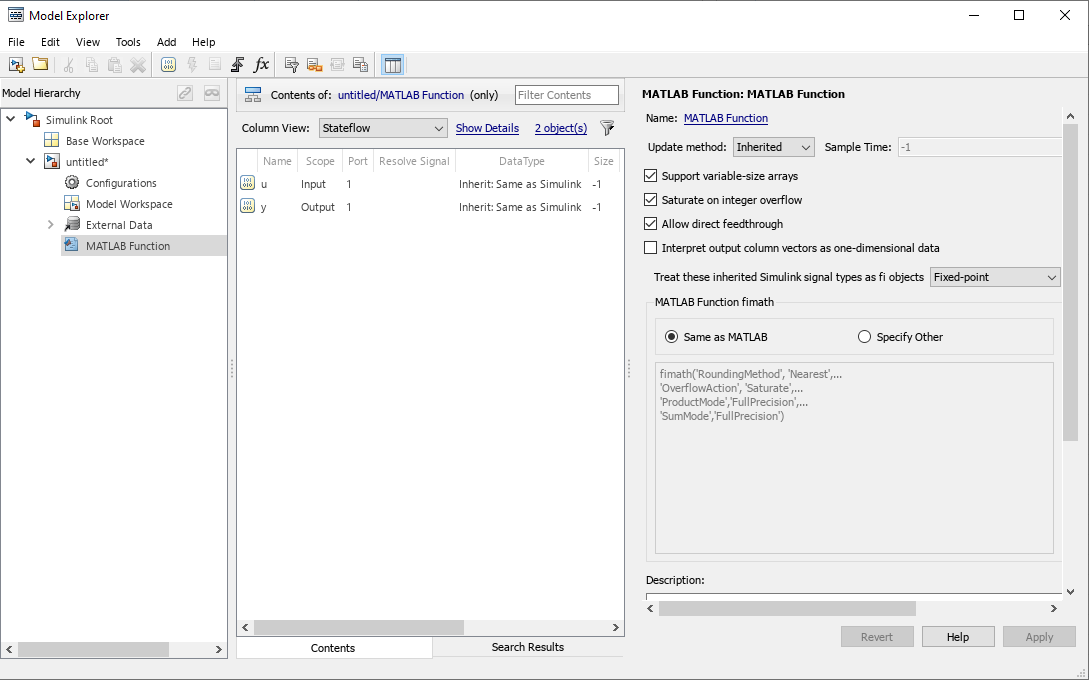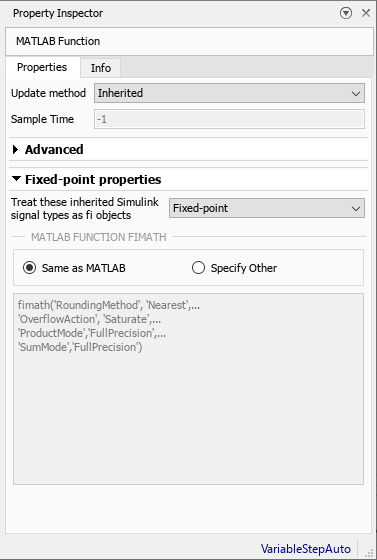## Control Data Types and Generate Code with MATLAB Function Block

You can use the MATLAB Function block to compose a MATLAB® language function in a Simulink® model that generates embeddable code. When you simulate the model or generate code for a target environment, a function in a MATLAB Function block generates efficient C/C++ code. This code meets the strict memory and data type requirements of embedded target environments.

### Data Type Override with MATLAB Function Block

When you use the MATLAB Function block in a Simulink model that specifies data type override, the block determines the data type override equivalents of the input signal and parameter types. The block then uses these equivalent values to run the simulation. This table shows how the MATLAB Function block determines the data type override equivalent using the data type of the input signal or parameter and the data type override settings in the Simulink model. For more information about data type override, see Fixed-Point Instrumentation and Data Type Override.

Input Signal or Parameter TypeData Type Override SettingData Type Override Applies To SettingOverride Data Type
Inherited `single``Double``All numeric types` or `Floating-point`Built-in `double`
`Single``All numeric types` or `Floating-point`Built-in `single`
`Scaled double``All numeric types` or `Floating-point``fi scaled double`
Specified `single``Double``All numeric types` or `Floating-point`Built-in `double`
`Single``All numeric types` or `Floating-point`Built-in `single`
`Scaled double``All numeric types` or `Floating-point``fi scaled double`
Inherited `double``Double``All numeric types` or `Floating-point`Built-in `double`
`Single``All numeric types` or `Floating-point`Built-in `single`
`Scaled double``All numeric types` or `Floating-point``fi scaled double`
Specified `double``Double``All numeric types` or `Floating-point`Built-in `double`
`Single``All numeric types` or `Floating-point`Built-in `single`
`Scaled double``All numeric types` or `Floating-point``fi scaled double`
Inherited `Fixed``Double``All numeric types` or `Fixed-point``fi double`
`Single``All numeric types` or `Fixed-point``fi single`
`Scaled double``All numeric types` or `Fixed-point``fi scaled double`
Specified `Fixed``Double``All numeric types` or `Fixed-point``fi double`
`Single``All numeric types` or `Fixed-point``fi single`
`Scaled double``All numeric types` or `Fixed-point``fi scaled double`

### Fixed-Point Data Types with MATLAB Function Block

Code generation from MATLAB supports a significant number of Fixed-Point Designer™ functions. For information about which Fixed-Point Designer functions are supported, see Functions Supported for Code Acceleration or C Code Generation. To simulate models using fixed-point data types in Simulink, you must have a Fixed-Point Designer license.

#### Specify Fixed-Point Parameters in the Model Explorer

You can use the Model Explorer to specify parameters for a MATLAB Function block in a fixed-point model. For more information, see Specify MATLAB Function Block Properties.

1. Create a new model. Place a MATLAB Function block in the model.

2. Open the Model Explorer. On the Modeling tab, in the Design section, click .

3. Expand the untitled* node in the Model Hierarchy pane. Then, select the MATLAB Function node.These parameters apply to MATLAB Function blocks in models that use fixed-point and integer data types:

• Treat these inherited Simulink signal types as fi objects — Choose whether to treat inherited fixed-point and integer signals as `fi` objects.

• `Fixed-point` — The MATLAB Function block treats all fixed-point inputs as Fixed-Point Designer `fi` objects.

• `Fixed-point & Integer` — The MATLAB Function block treats all fixed-point and integer inputs as Fixed-Point Designer `fi` objects.

• MATLAB Function fimath — Specify the `fimath` properties for the block to associate with these objects:

• All fixed-point and integer input signals to the MATLAB Function block that you choose to treat as `fi` objects.

• All `fi` and `fimath` objects constructed in the MATLAB Function block.

Select one of these options:

• Same as MATLAB — The block uses the same `fimath` properties as the current default `fimath`. The edit box displays the current default `fimath` in read-only form.

• Specify Other — Specify your own `fimath` object in the edit box.

#### Use `fimath` Objects in MATLAB Function Blocks

Open the Property Inspector pane for the MATLAB Function block. On the Modeling tab, in the Design section, select Property Inspector. In the Property Inspector pane, expand Fixed-point properties.The MATLAB Function block fimath parameter enables you to specify one set of `fimath` object properties for the MATLAB Function block. The block associates the `fimath` properties you specify with the following objects:

• All fixed-point and integer input signals to the MATLAB Function block that you choose to treat as `fi` objects.

• All `fi` and `fimath` objects constructed in the MATLAB Function block.

You can select one of the following options:

• Same as MATLAB — The block uses the same `fimath` properties as the current default `fimath`. The edit box displays the current default `fimath` in read-only form.

• Specify Other — Specify your own `fimath` object in the edit box. You can do this in two ways:

• Construct the `fimath` object inside the edit box.

• Construct the `fimath` object in the MATLAB or model workspace and then enter its variable name in the edit box.

Note

If you use this option and plan to share your model with others, make sure you define the variable in the model workspace.

For an example showing the MATLAB Function fimath options work, see Specify Fixed-Point Math Properties in MATLAB Function Block.

The Fixed-Point Designer `isfimathlocal` function supports code generation for MATLAB.

### Share Models Containing Fixed-Point MATLAB Function Blocks

To share a fixed-point model containing a MATLAB Function block, you must first move any variables you define in the MATLAB workspace, including `fimath` objects, to the model workspace. For example:

1. Create a new model. Place a MATLAB Function block in the model.

2. Define a `fimath` object in the MATLAB workspace.

```F = fimath('RoundingMethod','Floor','OverflowAction','Wrap',... 'ProductMode','KeepLSB','ProductWordLength',32,... 'SumMode','KeepLSB','SumWordLength',32)```
```F = RoundingMethod: Floor OverflowAction: Wrap ProductMode: KeepLSB ProductWordLength: 32 SumMode: KeepLSB SumWordLength: 32 CastBeforeSum: true```
You can use this `fimath` for any Simulink fixed-point signal entering the MATLAB Function block as an input.

3. Open the Model Explorer.

4. Expand the untitled* node in the Model Hierarchy pane of the Model Explorer. Select the MATLAB Function node.

5. For MATLAB Function block fimath, select Specify other. In the edit box, enter the variable `F`. Click to save your changes.

You have now defined the `fimath` properties to be associated with all Simulink fixed-point input signals and all `fi` and `fimath` objects constructed within the block.

6. In the Model Hierarchy pane, select Base Workspace. You can see the variable `F` that you have defined in the MATLAB workspace listed in the Contents pane. If you send this model to another user, that user must first define that same variable in the MATLAB workspace to get the same results.

7. Cut the variable `F` from the base workspace and paste it into the model workspace listed under the node for your model, in this case, untitled*. The Model Explorer now appears as shown.You can share your model with another user. Because you included the required variables in the workspace of the model itself, another user can run the model and get the correct results. Receiving and running the model does not require any extra steps.